# An introduction to Flowcharts

• Difficulty Level : Basic
• Last Updated : 20 Nov, 2020

What is a Flowchart?
Flowchart is a graphical representation of an algorithm. Programmers often use it as a program-planning tool to solve a problem. It makes use of symbols which are connected among them to indicate the flow of information and processing.
The process of drawing a flowchart for an algorithm is known as “flowcharting”.

Basic Symbols used in Flowchart Designs

Attention reader! All those who say programming isn't for kids, just haven't met the right mentors yet. Join the  Demo Class for First Step to Coding Coursespecifically designed for students of class 8 to 12.

The students will get to learn more about the world of programming in these free classes which will definitely help them in making a wise career choice in the future.

1. Terminal: The oval symbol indicates Start, Stop and Halt in a program’s logic flow. A pause/halt is generally used in a program logic under some error conditions. Terminal is the first and last symbols in the flowchart.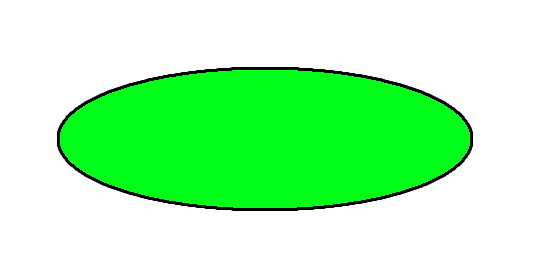• Input/Output: A parallelogram denotes any function of input/output type. Program instructions that take input from input devices and display output on output devices are indicated with parallelogram in a flowchart.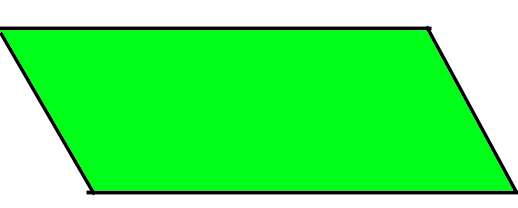• Processing: A box represents arithmetic instructions. All arithmetic processes such as adding, subtracting, multiplication and division are indicated by action or process symbol.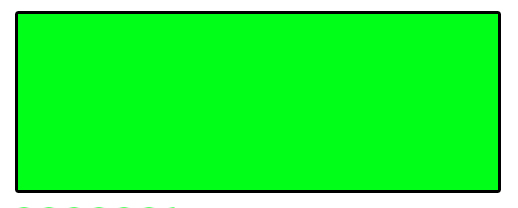• Decision Diamond symbol represents a decision point. Decision based operations such as yes/no question or true/false are indicated by diamond in flowchart.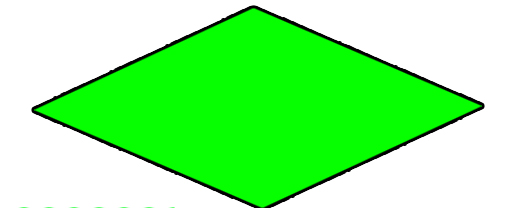• Connectors: Whenever flowchart becomes complex or it spreads over more than one page, it is useful to use connectors to avoid any confusions. It is represented by a circle.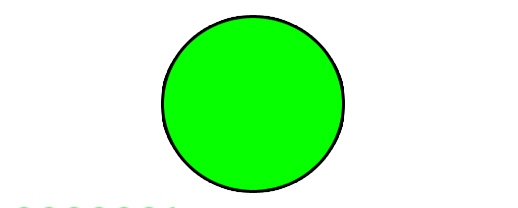• Flow lines: Flow lines indicate the exact sequence in which instructions are executed. Arrows represent the direction of flow of control and relationship among different symbols of flowchart.

• Flowcharts are better way of communicating the logic of system.
• Flowcharts act as a guide for blueprint during program designed.
• Flowcharts helps in debugging process.
• With the help of flowcharts programs can be easily analyzed.
• It provides better documentation.
• Flowcharts serve as a good proper documentation.

• It is difficult to draw flowchart for large and complex programs.
• In this their is no standard to determine the amount of detail.
• Difficult to reproduce the flowcharts.
• It is very difficult to modify the Flowchart.

Example : Draw a flowchart to input two numbers from user and display the largest of two numbers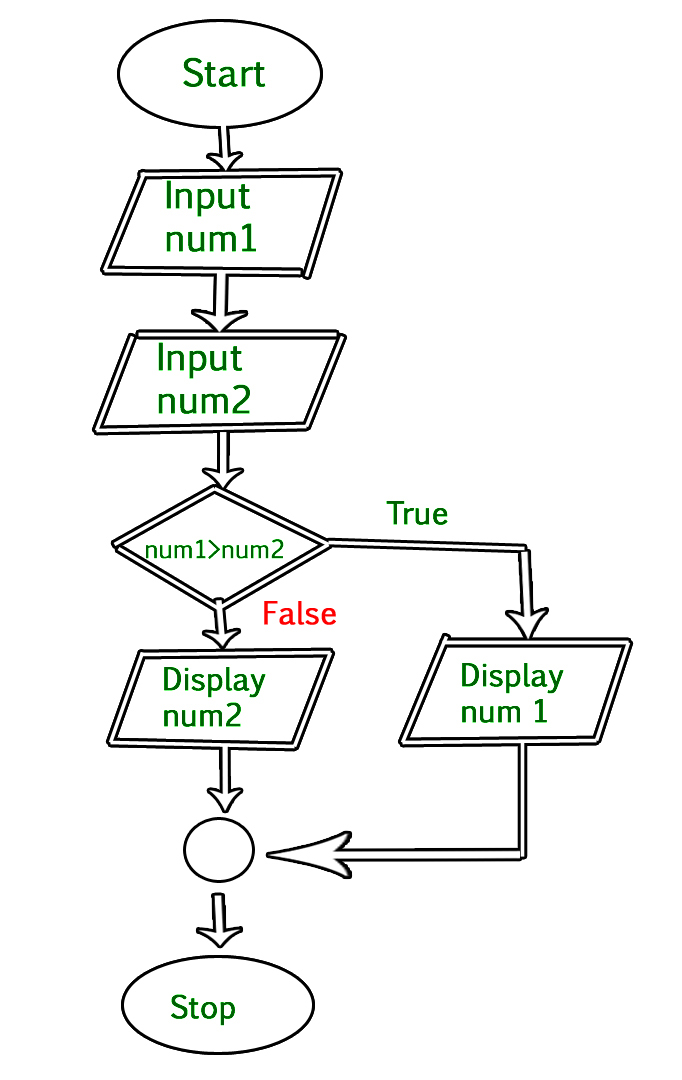## C

 `// C program to find largest of two numbers` `#include ` `int` `main()``{``    ``int` `num1, num2, largest;` `    ``/*Input two numbers*/``    ``printf``(``"Enter two numbers:\n"``);``    ``scanf``(``"%d%d"``, &num1, &num2);` `    ``/*check if a is greater than b*/``    ``if` `(num1 > num2)``        ``largest = num1;``    ``else``        ``largest = num2;` `    ``/*Print the largest number*/``    ``printf``(``"%d"``, largest);` `    ``return` `0;``}`

## C++

 `// C++ program to find largest of two numbers``#include ``using` `namespace` `std;``int` `main()``{``    ``int` `num1, num2, largest;` `    ``/*Input two numbers*/``    ``cout << ``"Enter two numbers:\n"``;``    ``cin >> num1;``    ``cin >> num2;` `    ``/*check if a is greater than b*/``    ``if` `(num1 > num2)``        ``largest = num1;``    ``else``        ``largest = num2;` `    ``/*Print the largest number*/``    ``cout << largest;` `    ``return` `0;``}`

## Java

 `// Java program to find largest of two numbers``import` `java.util.Scanner;``public` `class` `largest {``    ``public` `static` `void` `main(String args[])``    ``{``        ``int` `num1, num2, max;` `        ``/*Input two numbers*/``        ``Scanner sc = ``new` `Scanner(System.in);``        ``System.out.println(``"Enter two numbers:"``);` `        ``num1 = sc.nextInt();``        ``num2 = sc.nextInt();` `        ``/*check whether a is greater than b or not*/``        ``if` `(num1 > num2)``            ``max = num1;``        ``else``            ``max = num2;` `        ``/*Print the largest number*/``        ``System.out.println(max);``    ``}``}`

## C#

 `// C# program to find largest of two numbers``using` `System;``using` `System.IO;` `class` `GFG``{``    ``static` `public` `void` `Main ()``    ``{``        ` `        ``int` `num1, num2, max;` `        ``/*Input two numbers*/``        ``Console.WriteLine(``"Enter two numbers:"``);` `        ``num1 = Convert.ToInt32(Console.ReadLine());``        ``num2 = Convert.ToInt32(Console.ReadLine());` `        ``/*check whether a is greater than b or not*/``        ``if` `(num1 > num2)``            ``max = num1;``        ``else``            ``max = num2;` `        ``/*Print the largest number*/``        ``Console.WriteLine(max);``    ``}``}` `// This code is contributed by NamrataSrivastava1`

Output

```Enter two numbers:
10 30
30```

References:
Computer Fundamentals by Pradeep K. Sinha and Priti Sinha

My Personal Notes arrow_drop_up